# Methods of Differentiation | Concept Booster IIT-JEE Class 12 notes

• 1
•
•
•
• 4
•
•
•
5
Shares

## Methods of Differentiation

Here we will learn methods of Differentiation, We had already studied the two meanings of derivative(differentiation) of a function which is the geometrical and physical meanings. Since we know what does the differentiation of a function means and how it’s defined therefore now we will find derivatives of various functions using methods of differentiation. these methods of differentiation are useful as these help in finding derivatives of various functions.

### First and Second derivative of a function

When we talk about the derivative(differentiation) of a function we simply means first derivative denoted as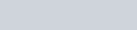it should be noted that the differential operator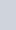must be seen as a single operator , but this first order differential coefficients are so much flexible that one can algebraically manipulate them like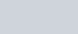But that’s not the case with higher order differential coefficients.

the second derivative of a function means the derivative of derivative of the function denoted as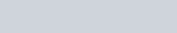Similarly higher order derivatives are defined, the second order differential coefficients should not be manipulated algebraically like we can do we the first order. this implies that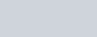### Some Standard Derivatives

Here some of the first derivatives of some functions are given which are useful in finding derivatives of various functions. Some Standard methods of differentiation are

### Some Standard Substitutions

For finding differentiation of any function the most prior thing is to simplify the given function and then differentiate it. there are some standard substitutions are used in certain situations where we are finding differentiation of the function

## Fundamental Theorems on Differentiation

Here we are listing some of the very fundamental theorems on differentiation , these theorems can be proved using the basic definition of differentiation , as per first principle of differentiation.

talking for any two functions f(x) and g(x) which are differentiable. the basic methods of differentiation are

#### Derivative of sum/difference of two functions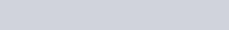#### Product Rule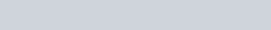#### Quotient Rule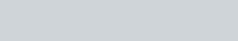#### Chain Rule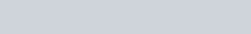## Important Points to Know

• if f(x) and g(x) are inverse of each other then f(g(x))=x, by definition of inverse function. in this case we obtain an important result as proceeded below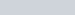differentiating both sides w.r.t x and applying chain rule we get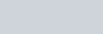• A equation whose degree of each term is “n” is said to be a homogenous equation of nth degree, for any homogenous equation the value of derivative will be always be given by,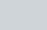we’ll look on it’s proof in the topic of differential equations.

## Differentiation of one function w.r.t Another function

If we have two functions in terms of a same variable, for example we have f(x) and g(x) , then the differentiation of f(x) with respect to g(x) is given as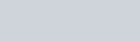## Differentiation of implicit function

a Function is defined to be an implicit function if “y” can’t be independently expressed in terms of x. and if the function is explicit if “y” can be independently expressed in terms of x. An Implicit function contains both variables (x,y) in the terms which can’t be separated. Hence Implicit function is denoted as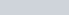.A Part of a circle highlighted is expressed by an implicit equation. File credits

there is a Result to directly obtain the differentiation of any implicit function.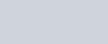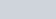denotes partial differentiation of the function with respect to variable “x”, which means differentiating the function with respect to “x”, while assuming “y” to be constant.

similarly,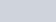denotes partial differentiation of the function with respect to variable “y”, which means differentiating the function with respect to “y”, while assuming “x” to be constant.

## Parametric Differentiation

When variables x and y are expressed in terms of any parameter let’s say both x and y are expressed in terms of “t” then the derivative of y with respect to x is evaluated as follows: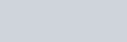## Differentiation of determinants

let’s we have a following determinant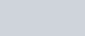the derivative of determinant is given by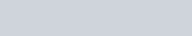and so on for any determinant the derivative is basically sum of determinants in such a way that first we differentiate first row while keeping others same in determinant and add it with the determinant whose second row is differentiated and other elements remains same, and so on. depending on number of rows.